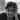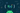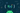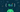Navigate back to the homepage

# Functional Programming in Kotlin - f(4)Hari Vignesh Jayapalan
May 23rd, 2020 · 2 min read## Currying Function

This is the fourth part of the functional programming series. If you have missed the previous one, start here.

### Series pit stops

In this pit stop, we’ll take a brief look at how we write or represent currying functions in Kotlin.

### Pre-requisites

• Basic programming knowledge
• School mathematics
• Previous pit stop

### Mathematical Currying

Since we talked just f(x), here x can be treated as an argument in programming perspective. So our examples before were only for functions with one argument. How do we compose for functions with multiple arguments?

In FP concept, a function cannot have multiple arguments. Shocked? don’t be. If you recall before, a function is just a map from one source set to another. It’s not a map from multiple source sets to target sets. But on the other hand, a product of 2 sets can result in a set. Such a product of sets, resulting a function which is a set, can resemble like a function of multiple arguments.

For example,

`.css-1chxjt6{position:absolute;right:22px;top:24px;padding:8px 12px 7px;border-radius:5px;color:#6f7177;-webkit-transition:background 0.3s ease;transition:background 0.3s ease;}.css-1chxjt6:hover{background:rgba(255,255,255,0.07);}.css-1chxjt6[data-a11y="true"]:focus::after{content:"";position:absolute;left:-2%;top:-2%;width:104%;height:104%;border:2px solid var(--theme-ui-colors-accent,#6166DC);border-radius:5px;background:rgba(255,255,255,0.01);}@media (max-width:45.9375em){.css-1chxjt6{display:none;}}1f(x, y) = 2x + y`

Here the element x is a pair of 2 elements. Let’s take it as 33 and 48.Here the function of 2 sets are called Tuple.

`1f((33, 48)) = 2x + y = 66 + 48 => 114`

Currying a function is nothing but an operation where we apply one function at a time, pass the result to the next and so on, till we reach the result. Let’s take the same example f(x, y) = 2x + y

This can also be written as

`1f(x)(y) = 2x + y`

Let’s consider f(x) as g. Then,

`1if f(x) = g, then2g(y) = 2x + y`

When f(x) is applied to be g, then the value x in 2x + y is a constant. It’s not a variable to be substituted with. Hence,

`1g(y) = 2(33) + y2g(y) = 66 + y = 66 + 48 => 114`

In other words, when we apply x for the function f, we get a resulting function g which is applied to y. This is process is called currying a function.

### Kotlin Currying

When we represent tuple in Kotlin (also NOT known as function with 2 parameters), we just pass the result to next function. Like

`1(Int) -> (Int) -> Int`

The above represents : A function, that takes an integer as a parameter (Int) and returns a function of (Int) -> Int

It’s time for some examples of currying in Kotlin. Let’s take the above functions and try to represent in Kotlin.

`1val currying: (Int) -> (Int) -> Int = 2{ x -> { y -> (2 * x) + y } }34println(currying(33)(48)) 56// output -> 114`

Now, like our previous pitstop - what if we write a generalized function that can curry two functions?

`1fun r1(x: Boolean): Int = if(x) 1 else 02fun r2(x: Int): Boolean = if(x == 0) false else true34fun <T, U, V>currying() = {f: (U) -> V -> { g: (T) -> U -> { h: T -> f(g(h)) }}}56val r1of2 = compose<Int, Boolean, Int>()(::r1)(::r2)78println(r1of2(2)) // output -> 1`

In short, currying is a process in functional programming in which we can transform a function with multiple arguments into a sequence of nesting functions. It returns a new function that expects the next argument inline. It keeps returning the function until it exhausts the arguments.

If you think you have learned something new or if you like this series or you want to boost my morale, please share the post.

### More articles from Hari Vignesh Jayapalan### Functional Programming in Kotlin - f(3)

Function Composition

May 14th, 2020 · 2 min read### Functional Programming in Kotlin - f(2)

Functions in Kotlin | Pure Functions

May 3rd, 2020 · 3 min read